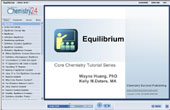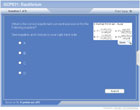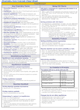How to Learn in 24 Hours?The Rapid Learning Movie

 Need Help? M-F: 9am-5pm(PST): Toll-Free: (877) RAPID-10 US Direct: (714) 692-2900 Int'l: 001-714-692-2900 24/7 Online Technical Support: The Rapid Support Center Secure Online Order:Need Proof? Testimonials by Our Users

 Rapid Learning Courses: MCAT in 24 Hours (2015-16) USMLE in 24 Hours (Boards) Chemistry in 24 Hours Biology in 24 Hours Physics in 24 Hours Mathematics in 24 Hours Psychology in 24 Hours SAT in 24 Hours ACT in 24 Hours AP in 24 Hours CLEP in 24 Hours DAT in 24 Hours (Dental) OAT in 24 Hours (Optometry) PCAT in 24 Hours (Pharmacy) Nursing Entrance Exams Certification in 24 Hours eBook - Survival Kits Audiobooks (MP3)

 Tell-A-Friend: Have friends taking science and math courses too? Tell them about our rapid learning system.

 FAIL (the browser should render some flash content, not this).Home »  Chemistry »  College Chemistry

Equilibrium

 Topic Review on "Title": Dynamic equilibrium is when the rate of the forward and reverse reactions are equal.  The reaction will appear to have stopped, as the concentrations of each species won’t change, but the reaction continues to proceed in both directions. Equilibrium constants Equilibrium constant expressions are the ratio of the concentrations of products to reactants using their coefficients from the balanced equation as exponents.  Pure solids and liquids are not included in the equilibrium constant expression.  Equilibrium concentrations are plugged into the expression to solve for the equilibrium constant.  A large equilibrium constant means that the reaction “lies to the right” with more products than reactants at equilibrium.  A small constant means that the reaction “lies to the left” with more reactants the products present at equilibrium. Reaction Quotients The reaction quotient is the value when concentrations at any time are plugged into the equilibrium constant expression.  If the reaction quotient equals the equilibrium constant, then the system is at equilibrium.  If the reaction quotient is too large, the reaction will proceed to the left to produce more reactants (and reduce products).  The opposite will happen if the reaction quotient is too small. Solving equilibrium problems A simple technique for solving equilibrium problems is the ICE chart method: Make a table with the reactants and products across the top Place “ICE” down the left hand side, for Initial, Change and Equilibrium. Fill in any given information from the problem. Use the balanced equation’s stoichiometric ratio to determine the “change” row. To solve the problem using the ICE chart: If an equilibrium concentration is known, you can determine the “change” and find the other equilibrium concentrations to plug in and solve for K If you don’t know any equilibrium concentrations, write expressions for them and plug in the expressions into your “K” equation to solve for them. If the K is very tiny (10-5 or smaller), you may approximate that the change is insignificant compared to the original value (if the original value is > 0)  e.g.:  0.25 M – x » 0.25 M Solubility equilibrium The solubility equilibrium constant is written for a dissolution reaction (a solid compound dissociates when dissolved in water).  The pure solid reactant is not included in the expression.  A saturated solution is one that is at equilibrium.  Le Chatelier’s Principle Le Chatelier’s principle says that if a system at equilibrium is stressed or changed, the system will shift to reach equilibrium again.  Whatever you do to the system, it will un-do it.  For example, if you add more reactants, the reaction will proceed to the right to get rid of the extra reactants and make more products.

Rapid Study Kit for "Title":
 Flash Movie Flash Game Flash Card Core Concept Tutorial Problem Solving Drill Review Cheat Sheet"Title" Tutorial Summary : Equilibrium is established when the rate of the forward reaction is equal to the rate of reaction of the reverse reaction in a reversible reaction.  This tutorial introduces how equilibrium is established, how equilibrium constant expressions are written and how equilibrium constants are calculated.  A problem solving technique for equilibrium problems will be illustrated in the examples.  The use of the reaction quotient to determine if a system is at equilibrium is illustrated.  Le Chatelier’s principle will be introduced, along with solubility equilibrium.

 Tutorial Features: Specific Tutorial Features: Molecular animation of establishment of dynamic equilibrium Animation of equations to show a change in the ratio of product to reactants Use of “ICE Chart” problem solving technique for solving equilibrium problems Application of Le Chatelier’s principle to the manufacturing industry  Series Features: Concept map showing inter-connections of new concepts in this tutorial and those previously introduced. Definition slides introduce terms as they are needed. Visual representation of concepts Animated examples—worked out step by step A concise summary is given at the conclusion of the tutorial.

 "Title" Topic List: Dynamic equilibrium Equilibrium constant Writing expressions Calculating constants Meaning of the equilibrium constant Reaction quotient Writing expressions and finding the value Using it to determine if it’s at equilibrium Solving equilibrium problems Using the ICE chart Solubility equilibrium Le Chatelier’s principle

See all 24 lessons in college chemistry, including concept tutorials, problem drills and cheat sheets:
Teach Yourself College Chemistry Visually in 24 Hours